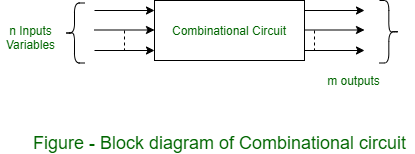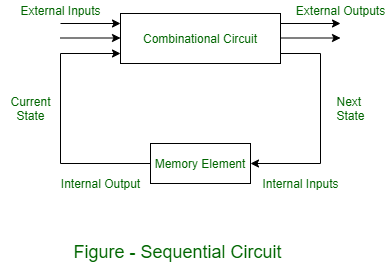Open in App
Not now

# Combinational and Sequential Circuits

• Difficulty Level : Easy
• Last Updated : 09 Jun, 2022

Digital logic circuits can be classified into “combinational” and “sequential”. A combinational logic circuit is one whose output solely depends on its current inputs. sequential circuits, on the other hand, are built using combinational circuits and memory elements called “flip-flops”. These circuits generate output that depends on the current and previous states. A Digital logic (or Switching) circuit has voltage levels to be switched from one value to another but has a finite number of distinct values (generally 0 for false and 1 for true). These circuits operate a definite set of logic rules so it is also known as Logic circuits. These are basic circuits that are used in mobile phones, calculators, computers, etc.

Types of Logic Circuits: There are two types of Digital circuits depending on their output and memory used:

```(i) Combinational circuit, and
(ii) Sequential circuit ```

A combinational circuit consists of logic gates whose outputs at any time are determined from only the present combination of inputs and they have no memory. A sequential circuit consists of logic gates whose outputs at any time are determined from both the present combination of inputs and previous output. That means sequential circuits use memory elements to store the value of previous output.

1. Combinational Circuits: These circuits are developed using AND, OR, NOT, NAND, and NOR logic gates. These logic gates are building blocks of combinational circuits. A combinational circuit consists of input variables and output variables. Since these circuits are not dependent upon previous input to generate any output, so are combinational logic circuits. A combinational circuit can have an n number of inputs and m number of outputs. In combinational circuits, the output at any time is a direct function of the applied external inputs.2. Sequential circuits: A sequential circuit is specified by a time sequence of inputs, outputs, and internal states. The output of a sequential circuit depends not only on the combination of present inputs but also on the previous outputs. Unlike combinational circuits, sequential circuits include memory elements with combinational circuits. Some examples are counters and shift registers.• The memory elements are circuits capable of storing binary information.
• The binary information stored in these memory elements at any given time defines the state of the sequential circuit at that time.
• The external output of a sequential circuit depends both on the present input and the previous output state.
• The next state of the memory elements also depends on the external input and the present state of the external output.
• Some sequential circuits may not contain combinational circuits, but only memory elements.

Generally, there are two types of storage elements used: Latches, and Flip-Flops. Storage elements that operate with signal levels (rather than signal transitions) are referred to as latches; those controlled by a clock transition are flip-flops.

Another major difference between the combinational and sequential circuits is the feedback mechanism.

My Personal Notes arrow_drop_up